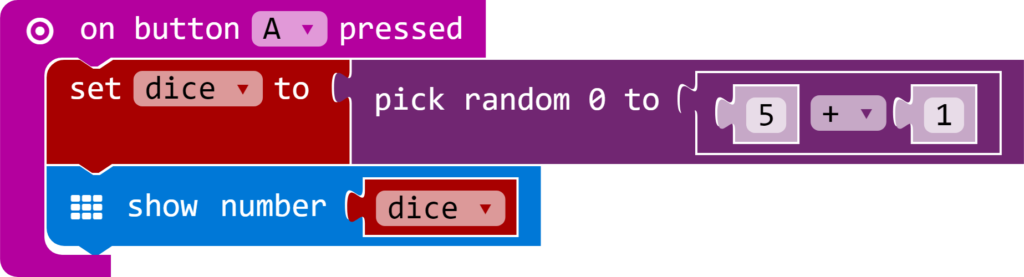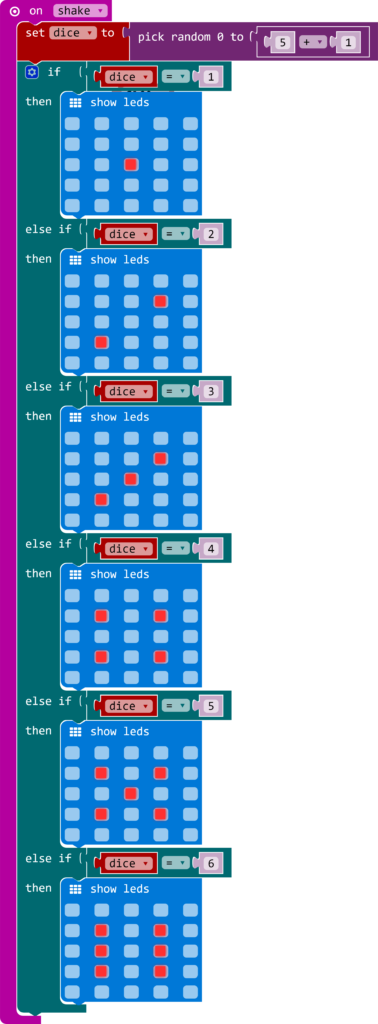Home Microbit microbit how to make a dice

# microbit how to make a dice

In this article we show you how to create a dice using your micro:bit, there are a couple of ways to do this and we will also show a couple of different development examples.

It is relatively straight forward to create a dice, we show two ways. The firs method displays a number on the led matrix and then we improve this by displaying a simulation of the actual dice spots on the led matrix.

For this first group of examples we will use the block editor and JavaScript at https://makecode.microbit.org/

In this first example we will simply display a random number from 1 to 6 on the micro:bit LED matrix, this is the blocks example. We will use the button A to trigger a roll of the dice, we create a variable called dice to store the number generated, since the random number generated is in the range 0 to 5 we simply add 1 to it. We then show that number on the led matrix using the show number block which takes the dice variable as its parameter

This is what you need to createIf you prefer JavaScript, here is the code for the example above – the editor make sit easy to switch between both

[codesyntax lang=”javascript”]

let dice = 0
input.onButtonPressed(Button.A, () => {
dice = Math.random(5 + 1 + 1)
basic.showNumber(dice)
})

[/codesyntax]

Of course a smarter way of doing this is rather than pressing a button to roll the dice, we shake the micro:bit as if we were physically rolling the dice – we will use the on shake block to do this. You need to create something like this

And again here is the JavaScript  code for the example above

[codesyntax lang=”javascript”]

let dice = 0
input.onGesture(Gesture.Shake, () => {
dice = Math.random(5 + 1 + 1)
basic.showNumber(dice)

})

[/codesyntax]

Now this is good so far but it is not really a dice is it, as we said at the start we associate the number of spots to match the number thrown. For the next example we will need to check the variable value using an if block with else if’s for each number.

For example if the number generated is 6 we want to display the number 6 via the show LEDS block.

You can see this in the complete example underneathAgain for the JavaScript fans, this is the code for the above example

[codesyntax lang=”javascript”]

```let dice = 0
input.onGesture(Gesture.Shake, () => {
dice = Math.random(5 + 1 + 1)
if (dice == 1) {
basic.showLeds(`
. . . . .
. . . . .
. . # . .
. . . . .
. . . . .
`)
} else if (dice == 2) {
basic.showLeds(`
. . . . .
. . . # .
. . . . .
. # . . .
. . . . .
`)
} else if (dice == 3) {
basic.showLeds(`
. . . . .
. . . # .
. . # . .
. # . . .
. . . . .
`)
} else if (dice == 4) {
basic.showLeds(`
. . . . .
. # . # .
. . . . .
. # . # .
. . . . .
`)
} else if (dice == 5) {
basic.showLeds(`
. . . . .
. # . # .
. . # . .
. # . # .
. . . . .
`)
} else if (dice == 6) {
basic.showLeds(`
. . . . .
. # . # .
. # . # .
. # . # .
. . . . .
`)
}
})
```

[/codesyntax]

We promised multiple languages so here we go

This next examples are written in python, it is back to one of the original examples where we shake the micro:bit and a random number is generated and we then display the number on the led matrix

We use the online Micropython editor option from https://www.microbit.co.uk/app/. You compile this and a hex file will be downloaded to your PC, you then drag this into your local drive that was created when you plugged in your micro:bit and this uploads the hex file to the device.

You should then be able to shake the device and see a pseudo random number appear.

[codesyntax lang=”python”]

```from microbit import *
import random
display.show('-')
while True:
if accelerometer.was_gesture('shake'):
display.clear()
sleep(1000)
display.show(str(random.randint(1, 6)))
sleep(10)

```

[/codesyntax]

Again the example does not really simulate a true dice, lets fix this in this python example

[codesyntax lang=”python”]

```from microbit import *
import random

one = Image("00000:"
"00000:"
"00900:"
"00000:"
"00000")

two = Image("00000:"
"09000:"
"00000:"
"00090:"
"00000")

three = Image("90000:"
"00000:"
"00900:"
"00000:"
"00009")

four = Image("00000:"
"09090:"
"00000:"
"09090:"
"00000")

five = Image("00000:"
"09090:"
"00900:"
"09090:"
"00000")

six = Image("09090:"
"00000:"
"09090:"
"00000:"
"09090")

display.scroll('Shake me!')

while True:
if accelerometer.was_gesture('shake'):
throw = random.randint(1, 6)
if throw == 1:
display.show(one)
if throw == 2:
display.show(two)
if throw == 3:
display.show(three)
if throw == 4:
display.show(four)
if throw == 5:
display.show(five)
if throw == 6:
display.show(six)
```

[/codesyntax]

Again download this to your PC, copy it to your micro:bit and there we go we now have a better dice example written in python

#### You may also like

This website uses cookies to improve your experience. We'll assume you're ok with this, but you can opt-out if you wish. Accept Read More